Next: Graphical solution of 2-var Up: The LP formulation and Previous: A prototype LP problem:

#### The general LP formulation

Generalizing formulation 5, the general form for a Linear Programming problem is as follows:

Objective Function: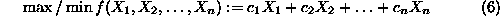s.t.
Technological Constraints: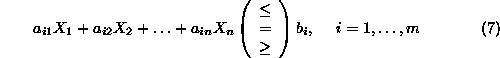Sign Restrictions: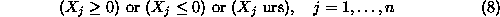where ``urs'' implies unrestricted in sign.

The formulation of Equations 6 to 8 has the general structure of a mathematical programming problem, presented in the introduction of this section, but it is further characterized by the fact that the functions involved in the problem objective and the left-hand-side of the technological constraints are linear. It is the assumptions implied by linearity that to a large extent determine the applicability of the above model in real-world applications.

To provide a better feeling of the linearity concept, let us assume that the different decision variables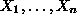correspond to various activities from which any solution will be eventually synthesized, and the values assigned to the variables by any given solution indicate the activity level in the considered plan(s). For instance, in the above example, the two activities are the production of items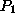and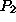, while the activity levels correspond to the daily production volume. Furthermore, let us assume that each technological constraint of Equation 7 imposes some restriction on the consumption of a particular resource. Referring back to the prototype example, the two problem resources are the daily production capacity of the two workstations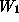and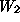. Under this interpretation, the linearity property implies that:

the total consumption of each resource, as well as the overall objective value are the aggregates of the resource consumptions and the contributions to the problem objective, resulting by carrying out each activity independently, and
Proportionality assumption:
these consumptions and contributions for each activity are proportional to the actual activity level.

It is interesting to notice how the above statement reflects to the logic that was applied when we derived the technological constraints of the prototype example: (i) Our assumption that the processing of each unit of product at every station requires a constant amount of time establishes the proportionality property for our model. (ii) The assumption that the total processing time required at every station to meet the production levels of both products is the aggregate of the processing times required for each product if the corresponding activity took place independently, implies that our system has an additive behavior. It is also interesting to see how the linearity assumption restricts the modeling capabilities of the LP framework: As an example, in the LP paradigm, we cannot immediately model effects like economies of scale in the problem cost structure, and/or situations in which resource consumption by one activity depends on the corresponding consumption by another complementary activity. In some cases, one can approach these more complicated problems by applying some linearization scheme. The resulting approximations for many of these cases have been reported to be quite satisfactory.

Another approximating element in many real-life LP applications results from the so called divisibility assumption. This assumption refers to the fact that for LP theory and algortihms to work, the problem variables must be real. However, in many LP formulations, meaningful values for the levels of the activities involved can be only integer. This is, for instance, the case with the production of itemsandin our prototype example. Introducing integrality requirements for some of the variables in an LP formulation turns the problem to one belonging in the class of (Mixed) Integer Programming (MIP). The complexity of a MIP problem is much higher than that of LP's. Actually, the general IP formulation has be shown to belong to the notorious class of NP-complete problems. (This is a class of problems that have been ``formally'' shown to be extremely ``hard'' computationally). Given the increased difficulty of solving IP problems, sometimes in practice, near optimal solutions are obtained by solving the LP formulation resulting by relaxing the integrality requirements - known as the LP relaxation of the corresponding IP - and (judiciously) rounding off the fractional values for the integral variables in the optimal solution. Such an approach can be more easily justified in cases where the typical values for the integral variables are in the order of tens or above, since the errors introduced by the rounding-off are rather small, in a relative sense.

We conclude our discussion on the general LP formulation, by formally defining the solution search space and optimality. Specifically, we shall define as the feasible region of the LP of Equations 6 to 8, the entire set of vectors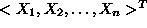that satisfy the technological constraints of Eq. 7 and the sign restrictions of Eq. 8. An optimal solution to the problem is any feasible vector that further satisfies the optimality requirement expressed by Eq. 6. In the next section, we provide a geometric characterization of the feasible region and the optimality condition, for the special case of LP's having only two decision variables.Next: Graphical solution of 2-var Up: The LP formulation and Previous: A prototype LP problem:

UAL Data
Fri Jun 20 15:03:05 CDT 1997